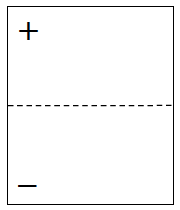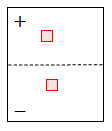### Home > CCA > Chapter A > Lesson A.1.4 > ProblemA-43

A-43.

Can zero be represented by any number of tiles? Using only the unit tiles (only the $1$ and $–1$ tiles), determine if you can represent zero on an Expression Mat with the number of tiles below. If you can, draw an Expression Mat demonstrating that it is possible. If it is not possible, explain why not.1.  $2$ tiles

Two identical tiles in opposite regions will make zero.There are other correct solutions.

1.  $6$ tiles

• In part (a), we reasoned that it is possible to make zero with $2$ tiles. Since $6$ is a multiple of $2$, we should also be able to make zero with $6$.

1.  $3$ tiles

Could you add a single tile to part (a) and still have zero?

Use the eTool below to solve the problem.
Click the link to the right for full version. CCA A-43 HW eTool